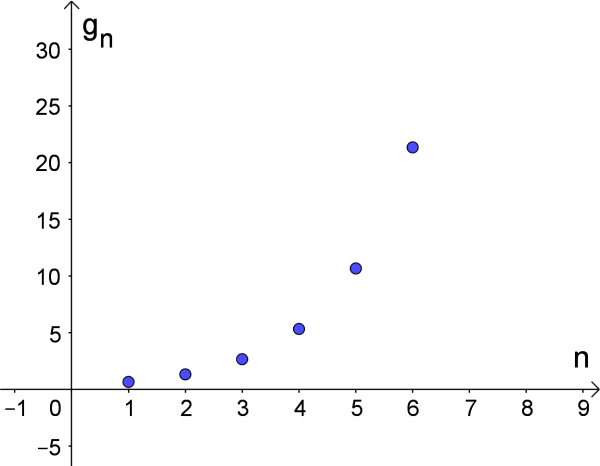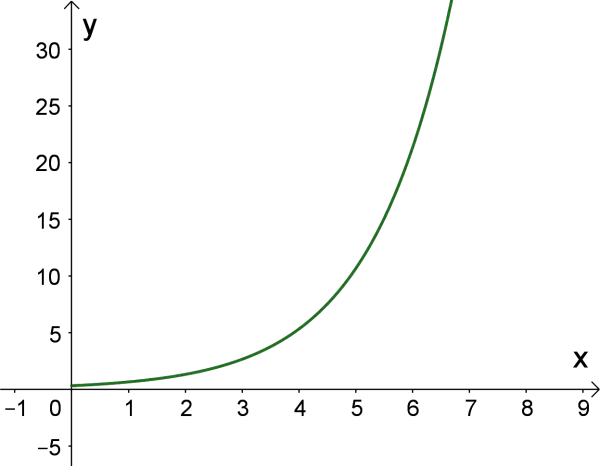# Geometric Progression Calculator

Number of Terms
First Term
Common Ratio
n^{th} Term  =  1024
n^{th} Partial Sum  =  2046
GENERATE WORK

## Geometric Progression - work with steps

Input Data :
Number of Terms (n) = 10
First Term (a) = 2
Common Ratio (r) = 2

Objective :
Find the n^{th} term & n^{th} partial sum of geometric series?

Formula :
n^{th} Term (T_n) = a x r(n - 1)
n^{th} Partial Sum (S_n) = a \times (r^n - 1)/(r - 1)

Solution :
n^{th} Term (T_n) = 2 x 2(10 - 1)
= 2 x 2(9)
= 2 x 512
n^{th} Term = 1024

S_n = (2(2^10 - 1))/(2 - 1)
 = (2(1024 - 1))/(1)
 = (2(1023))/(1)
 = (2046)/(1)
n^{th} Partial Sum = 2046

Geometric progression calculator calculates the $n^{th}$ term and the $n^{th}$ partial sum of a geometric progression.
It is necessary to follow the next steps:

1. Enter the number of terms of a geometric progression in the box. This value must be positive integer.
2. Enter the first term and the common ratio in the box. The first term can be a real number or variable, and the common ratio must be nonzero real number;
3. Press the "Generate Work" button to make the computation;
4. Geometric progression calculator will give $n^{th}$ term and the $n^{th}$ partial sum of a geometric progression.
Input: There are three inputs: the number of terms, the first term, and the common ratio of a geometric progression. The number of terms must be positive integer, the first term can be in terms of real numbers or variables, and the common ratio must be nonzero real number
Output: The outputs (the $n^{th}$ term and the sum of $n$ terms of the geometric progression) are in terms of nonzero real numbers of variables

$n^{th}$ term of a geometric progression:
• Explicit Formula: The $n^{th}$ term, $g_n$, of a geometric progression $(g_n )_{n\in N}$ is
$$g_n =g_1r^{n-1},\;\mbox{for}\;n\geq2$$
where $r\ne 0$ is the common ratio and $g_1$ is the initial term of the geometric progression.
• Recurrent Formula: The $n^{th}$ term of a geometric progression $(g_n )_{n\in N}$ is
$$g_n =g_{n-1}r,\;\mbox{for}\;n\geq2$$
where $r\ne 0$ is the common ratio and $g_{n-1}$ is the $(n-1)^{th}$ term of the geometric progression.
Formula for the n^{th} partial sum of a geometric progression:
The sum of the first $n$ terms, $S_n$, of a geometric progression $(g_n )_{n\in N}$:
$$S_n=\left\{ \begin{array}{ll} \frac{g_1(1-r^n)}{1-r}, & r\ne1; \\ n g_1, & r=1 \end{array} \right.$$ where $g_1$ and $r$ are is the initial term and the common ratio of the geometric progression, respectively, and $n$ number of terms in the geometric progression.

## What is Geometric Progression?

A geometric progression $(g_n )_{n\in N}$, or geometric sequence, is a sequence of real numbers or variables where each term is obtained from the preceding one by multiplying by a nonzero real number. The first term of a geometric progression is denoted by $g_1$, the second term by $g_2$, and so on up, the $n^{th}$ term by $g_n$. This means, any geometric progression $(g_n )_{n\in N}$ has the following form

$$g_1, g_1r, g_1r^2, g_1r^3, g_1r^4,\ldots$$

where the nonzero constant $r$ is the common ratio. The first term $g_1$ is called the initial term. Note that the common ratio $r$ cannot be zero. On the other hand, the progression $(g_n )_{n\in N}$ is a geometric progression with common ratio $r$ if the ratios between consecutive terms are equal, i.e.
$$\frac{g_2}{g_1}=\frac{g_3}{g_2}=\ldots=\frac{g_n}{g_{n-1}} =r$$

• If $r>1$, then the geometric progression is an increasing progression and it holds
$$g_1\lt g_2\lt \ldots\lt g_{n-1}\lt g_n$$
• If $0\lt r\lt 1$, then the geometric progression is an decreasing progression and it holds
$$g_1>g_2>\ldots>g_{n-1}>g_n$$
• If $r=1$, then the geometric progression is a constant progression and it holds
$$g_1=g_2=\ldots=g_{n-1}=g_n$$ The constant progression is only progression that is both geometric and geometric.
The terms between two nonconsecutive terms of a geometric progression $(g_n )_{n\in N}$ are called geometric means of these terms. For example, the geometric means between $g_1$ and $g_5$ are $g_2$, $g_3$ and $g_5.$ If two nonconsecutive terms of a geometric progression $(g_n )_{n\in N}$ and the number of geometric means between them are given, then the geometric progression is fully determined.
A geometric series is a sum of the terms of a geometric progression. A geometric series can be infinite or finite. The $n^{th}$ partial sum, usually denoted by the symbol $S_n$, represents the sum of the first $n$ terms of a series. This means that the geometric series is the following sum $$S_n=g_1+g_1r+g_1r^2+\ldots+g_1r^{n-1},$$ where $g_1$ is the initial term and $r\ne0$ is the common ratio.

### How to Calculate $n^{th}$ Term or Sum of $n$ Terms of Geometric Progression?

The $n^{th}$ term of geometric progression $(g_n )_{n\in N}$ can be defined recursively. By definition, the $n^{th}$ term $g_n$ is equal to $g_{n-1}r$, where $g_{n-1}$ is the $(n-1)^{th}$ term and $r$ is the common ratio. Therefore, $$g_n=g_{n-1}r$$ Since successive terms of a geometric progression can be determined as the product of the common ratio $r$ and the previous term, it follows that each term can be determined as the product of $g_1$ and a corresponding power of $r$. The formula for the $n^{th}$ term of a geometric progression $(g_n )_{n\in N}$ is $$g_n=g_{n-1}\cdot r=g_{n-2}\cdot r\cdot r=\ldots=g_{1}\cdot\underset{n-1}{\underbrace{r\cdot r\cdot\ldots\cdot r}}=g_1r^{n-1}$$ Hence, the $n^{th}$ term of a geometric progression can also be determined from the first term $g_1$ and the common ratio $r$. Any two terms $g_n$ and $g_m$ $(n>m>0)$ of a geometric progression $(g_n )_{n\in N}$ are related by the formula $$g_n=g_mr^{n-m}$$ In developing a formula for the $n^{th}$ partial sum for a finite geometric series, the series can be written in the following way $$S_n=g_1+g_1r+g_1r^2+\ldots+g_1r^{n-1}$$ On the other hand, by multiplying the previous equation by $r\ne 0$, we obtain $$S_n\cdot r =g_1r+g_1r^2+g_1r^3+\ldots+g_1r^{n-1}+g_1r^{n}$$ By substituting the last two equations, we obtain the formula for the sum of the first $n$ terms of a geometric progression with first term $g_1$ and common ratio $r$: $$S_n=\left\{ \begin{array}{ll} \frac{g_1(1-r^n)}{1-r}, & r\ne1; \\ n g_1, & r=1 \end{array} \right.$$ If a series has infinite number of terms, it is an infinite series. The sum of first $n$ terms of an infinite series is the $n^{th}$ partial sum of the series, $S_n$. In the following way, we can check whether the infinite geometric series converges or diverges.

• If $|r|<1$, the series converges and we can find its sum. It's sum is a finite number.
• If $|r|>1$, the series diverges and we can not find its sum which means that it's sum is not a finite number.
Geometric progression work with steps shows the complete step-by-step calculation for finding the $n^{th}$ term and the $n^{th}$ partial sum of a geometric progression such that there is $5$ terms in the geometric progression, the first term is $2$, and the common ratio is $4$. For any other combinations the number of terms, the first term, and the common ratio, just supply the other numbers as inputs and click on the on the "GENERATE WORK" button. The grade school students may use this geometric progression calculator to generate the work, verify the results or do their homework problems efficiently.

### Real World Problems Using Geometric Progression

A widespread application of geometric progression can be found in financial mathematics. Banks or financial companies usually use geometric progression to determine earnings in accounts or how much to charge for loans. For example, if we deposit $\$1 00$at the bank and bank offers an annual return of$3\%$of the investment, the deposited sum year increase by$3\%$after each year. The balances at the end of each year represent a geometric progression. The$n^{th}$term of this progression is$g_n=1.03g_{n-1}$and the initial term is$g_1=100$. Geometric progression can represent growth or decay. • If a common ratio$r$is greater than$1$,$r>1$, then a geometric progression may model growth. For instance, population growth. • If a common ratio$r$is positive and less than$1$,$0\lt r\lt 1$, then a geometric progression may model decay. For example, suppose that a new car loses one-fifth of its value each year. What is the value of this car after 3 years, if it costs now$\$30,0000$.
Geometric progression and exponential function are closely related. The difference between these two concepts is that a geometric progression is discrete while an exponential function is continuous function. For example, if we consider the geometric sequence $g_n=\frac{1}{3}\cdot {2}^n$ and the exponential function $f(x)=\frac{1}{3}\cdot 2^x,\;x>0,$ we obtain the following graphs:### Geometric Progression Practice Problems

Practice Problem 1:
We have $\$12$and go to the bank to deposit money. The bank gives us the following option: the first month we receive$\$18$, the second month we receive $\$27$, etc. How much money will we receive after$10$months? Practice Problem 2: Given the sequence by the recurrence relation$g_{n+1}=6g_n$and$g_1=3$. Find the sum of the first$10$terms of the geometric sequence. The geometric progression calculator, formulas for the$n^{th}$term of the geometric sequence and the sum of$n\$ numbers of the geometric sequence, example calculation (work with steps), real world problems and practice problems would be very useful for grade school students (K-12 education) in studying series and sequences and in solving problems in banking, biology and other real life fields.